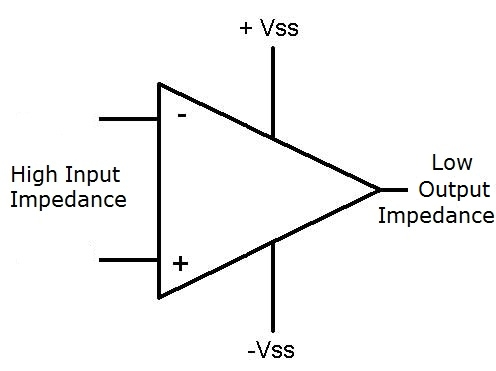﻿ Why Does an Op Amp Have a High Input Impedance and a Low Output Impedance?Why Does an Op Amp Have a High Input Impedance and a Low Output Impedance?Op Amp is a Voltage Gain Device

Op amps have high input impedance and low output impedance because of the concept of a voltage divider, which is how voltage is divided in a circuit depending on the amount of impedance present in given parts of a circuit.

Op amps are voltage gain devices. They amplify a voltage fed into the op amp and give out the same signal as output with a much larger gain. In order for an op amp to receive the voltage signal as its input, the voltage signal must be dropped across the op amp.

If you know the concept of a voltage divider, voltage drops primarily across components with high impedances, proportionally according to ohm's law by the formula V=IR. So the greater the resistance (or impedance) of a device, the greater the voltage drop across that device is. To make sure that the voltage signal drops fully on the op amp, it must have a very high input impedance, so that the voltage drops fully across it. If it had a low input impedance, the voltage may not drop across it and it would not receive the signal. This is why op amps must have high-input impedances.

For the same reason of a voltage divider, an op amp needs a low output impedance. Once the voltage is dropped across the op amp and it does its task of amplifying the signal, the signal should get dropped across the device that the op amp should feed. For example, say we have a microphone circuit. The signals spoken into the microphone need amplification from an op amp so that they can reach a level that can drive speakers. When the signals are amplified now, they are to be dropped across the speakers. Thus, the speakers must be of greater impedance than the output of the op amp, so that the voltage signals will drop across the speakers. Thus, the op amp needs a very low output impedance, so that the amplified voltage signal drops across the speakers and not the op amp. If not, the the amplified voltage signals will fall across the output impedance of the op amp and only partially on the speakers. The speakers will barely receive any signal and, thus, will not play back or output anything. This is way, op amp needs low output impedances, so that it can drop its amplified voltage signals across the desired device effectively and not on itself.